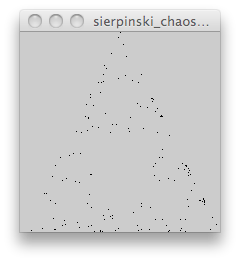# What is an Algorithm?

It’s simple: an algorithm is a set of steps! For example, here’s an algorithm to make a peanut butter and jelly sandwich:

1. Get a piece of bread
2. Spread peanut butter on it
3. Get another piece of bread
5. Put the two pieces of bread together
6. Eat it!

But is it really so simple? Where’s the bread? How do you “spread”? How do you put the two pieces of bread together?

You and I know how to do these things, but try telling a computer how to make a sandwich! When you write an algorithm for a computer, you have to be very specific, and you have to speak the computer’s language. You do this by using a programming language.

## The Chaos Game

Here’s an algorithm that creates a mathematical object called a fractal. Try this one on paper.

1. Draw a triangle and label the three vertices: 1 or 2, 3 or 4, 5 or 6
2. Randomly select a point inside the triangle to be your current position
3. Randomly select a vertex by rolling a six-sided die
4. Move half the distance from your current position to the selected vertex
5. Draw a dot here: this is your new current position
6. Repeat from step 3

Notice how step 6 says “Repeat”? In computer programming, this is called a loop. What happens if you repeat the loop in this algorithm 10 times? What about 50 times? 200 times?

OK, so maybe you were patient enough to draw 200 dots.

Congratulations! You’ve probably begun to see the fractal known as the Sierpinski triangle! What if you wanted to see this fractal with 1,000 dots? Or 10,000? You probably wouldn’t want to draw all of those dots by hand.

This is a great time to use the computer, which is really good at repeating simple things like this algorithm!

## The Chaos Game in Processing

There are many different programming languages out there. A great one to start with is called Processing, because it runs on any kind of computer, is free, and is easy to learn. Go to http://processing.org to download it. Then copy and paste in the program below, and hit the play button!

``````
// The Chaos Game; Sierpinski Triangle Generator

PVector[] vertices = new PVector;
PVector currentPosition;

void setup() {

size(200, 200);

// initialize the three vertices of the triangle
vertices = new PVector(width/2, 0);
vertices = new PVector(width, height);
vertices = new PVector(0, height);

// randomly select a point inside the triangle to be the current position
currentPosition = new PVector(random(0, width), random(0, height));

// draw the current position
point(currentPosition.x, currentPosition.y);
}

void draw() {

// draw the vertices
point(vertices.x, vertices.y);
point(vertices.x, vertices.y);
point(vertices.x, vertices.y);

// randomly select a vertex
int selectedVertex = int(random(0, 3));

// move half the distance from the current position to the selected vertex
currentPosition.x = (vertices[selectedVertex].x + currentPosition.x) / 2.0;
currentPosition.y = (vertices[selectedVertex].y + currentPosition.y) / 2.0;

// draw the current position
point(currentPosition.x, currentPosition.y);

}
``````Sierpinski Triangle in progress…

Brought to you by Nathan Selikoff, Wikipedia (Algorithm, Sierpinski triangle), and The Chaos Cookbook by Joe Pritchard